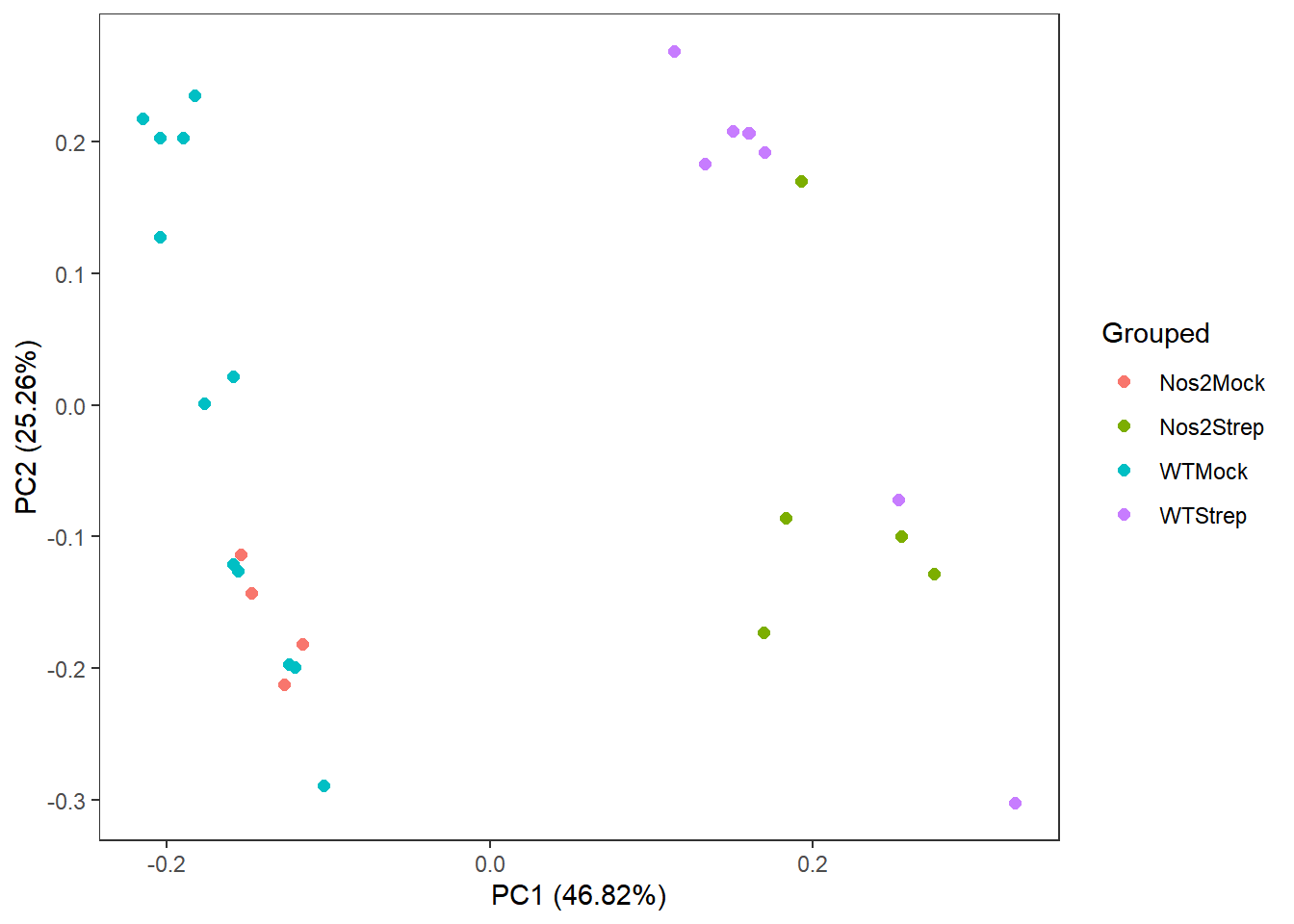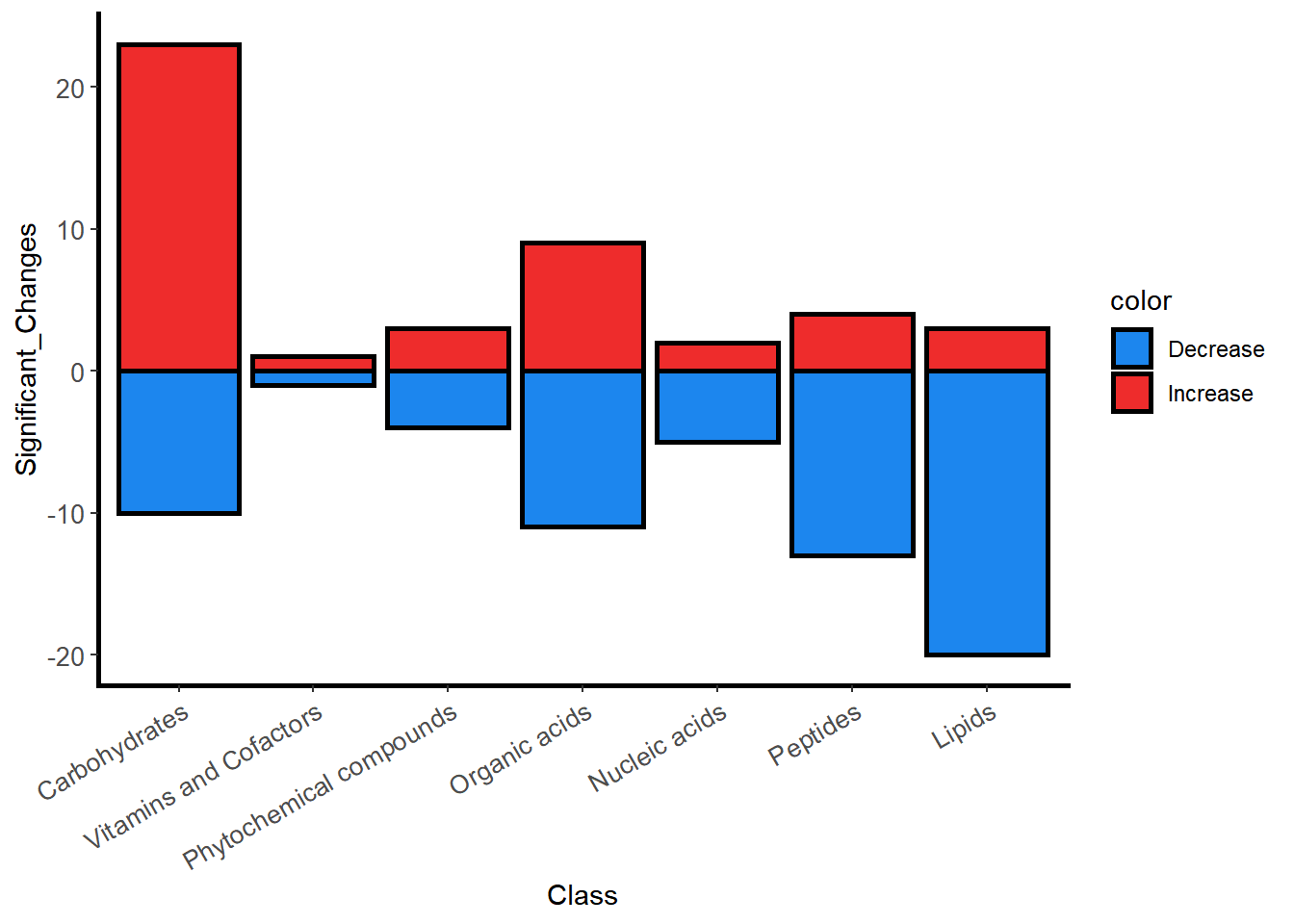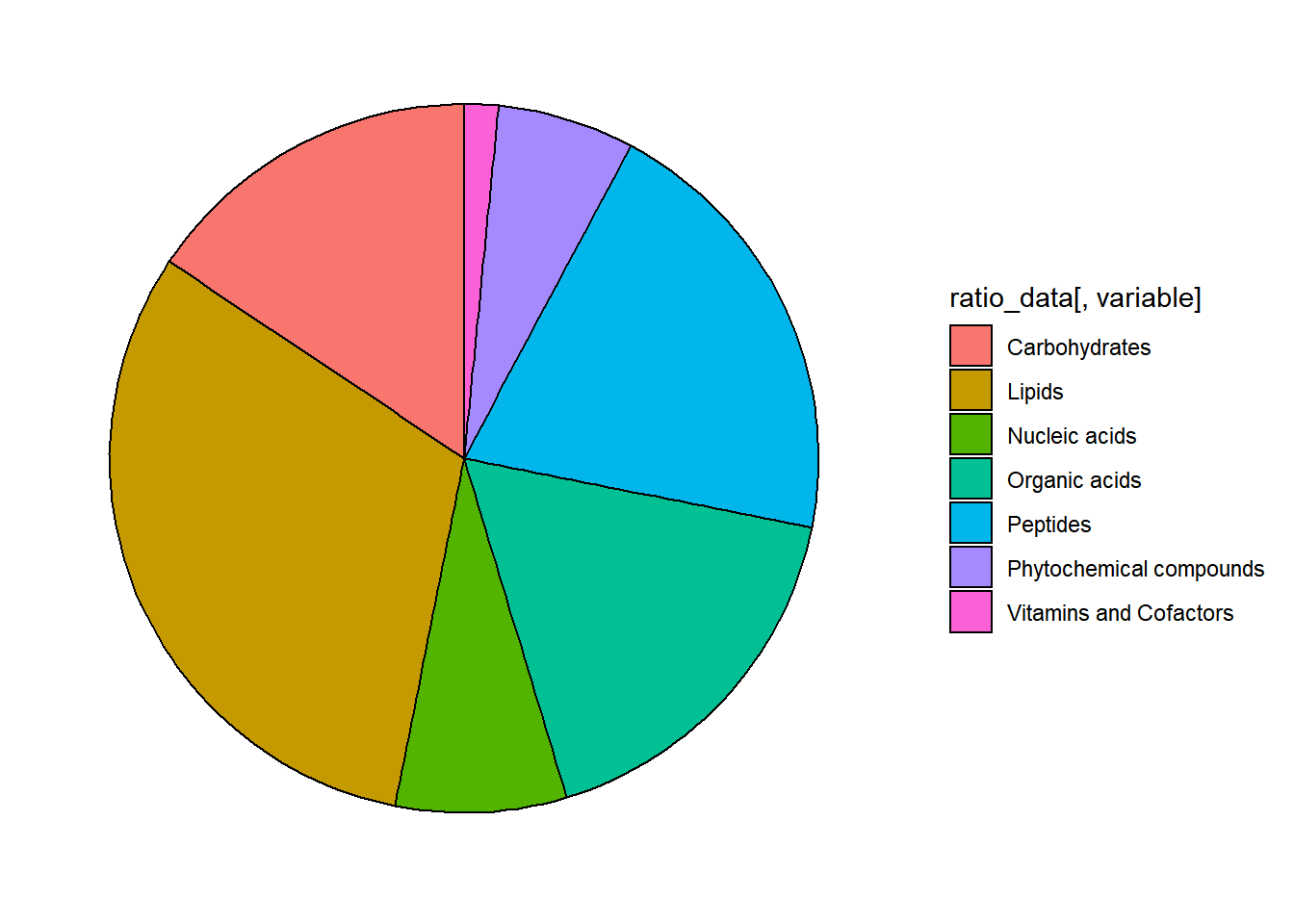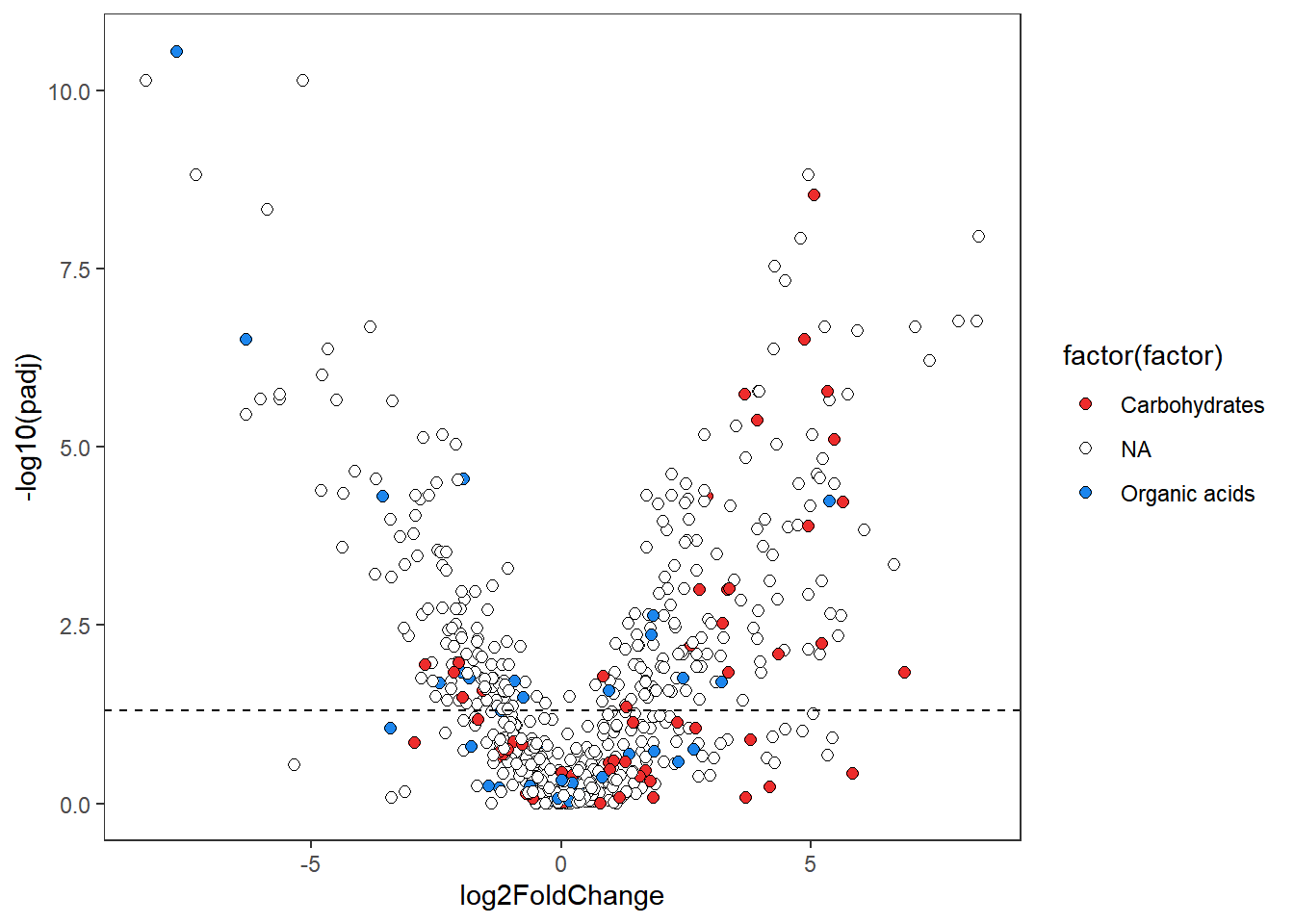## Introduction to omu

Omu is an R package that enables rapid analysis of Metabolomics data sets, and the creation of intuitive graphs. Omu can assign metabolite classes (Carbohydrates, Lipids, etc) as meta data, perform t tests, anovas and principle component analysis, and gather functional orthology and gene names from the KEGG database that are associated with the metabolites in a dataset. This package was developed with inexperienced R users in mind.

If your data do not yet have KEGG compound numbers you can acquire them by using the chemical translation service provided by the Fiehn lab here http://cts.fiehnlab.ucdavis.edu/

## Data Analysis

### Data Format

Included with Omu is an example metabolomics dataset of data from fecal samples collected from a two factor experiment with wild type c57B6J mice and c57B6J mice with a knocked out nos2 gene, that were either mock treated, or given streptomycin(an antibiotic), and a metadata file. To use Omu, you need a metabolomics count data frame in .csv format that resembles the example dataset, with the column headers Metabolite, KEGG, and then one for each of your samples. Row values are metabolite names in the Metabolite column, KEGG cpd numbers in the KEGG column, and numeric counts in the Sample columns.Additionally, for statistical analysis your data should already have undergone missing value imputation(eg. using random forest, k nearest neighbors, etc.). Here is a truncated version of the sample data in Omu as a visual example of this:

Metabolite KEGG C289_1
xylulose_NIST C00312 2424
xylose C00181 56311
xylonolactone_NIST C02266 637
xylonic_acid C00502 545

The meta data file should have a Sample column, with row values being sample names, and then a column for each Factor in your dataset, with row values being groups within that factor. Here is a truncated version of the metadata that accompanies the above dataset:

Sample Background Treatment Grouped
C289_1 WT Mock WTMock
C289_2 WT Mock WTMock
C289_3 WT Mock WTMock
C289_4 WT Mock WTMock

### Getting Your Data into R

For end users metabolomics data, it is recommended to use the `read.metabo` function to load it into R. This function is simply a wrapper for `read.csv`, which ensures your data has the proper class for `KEGG_gather` to work. For metadata, `read.csv` should be used.

``````your_metabolomics_count_dataframe <- read.metabo(filepath = "path/to/your/data.csv")

### Assiging Hierarchical Class Data

Omu can assign hierarchical class data for metabolites, functional orthologies, and organism identifiers associated with gene names. It does this using data frames located in the system data of the package(these can not be viewed or edited by the user, but the tables are available on the Omu github page in .csv format). To assign hierarchical class data, use the `assign_hierarchy` function and pick the correct identifier, either “KEGG”, “KO_Number”, “Prokaryote”, or “Eukaryote”. For example, using the c57_nos2KO_mouse_countDF.RData that comes with the package, compound hierarchy data can be assigned with the following code:

``DF <- assign_hierarchy(count_data = c57_nos2KO_mouse_countDF, keep_unknowns = TRUE, identifier = "KEGG")``

The argument `keep_unknowns = TRUE` keeps compounds without KEGG numbers, and compound hierarchy data was assigned by providing “KEGG” for the `identifier` argument. The output DF should look like this:

Metabolite KEGG Class Subclass_1 Subclass_2 Subclass_3 Subclass_4
xylulose_NIST C00312 Carbohydrates Monosaccharides Ketoses none none
xylose C00181 Carbohydrates Monosaccharides Aldoses none none
xylonolactone_NIST C02266 Carbohydrates Lactones none none none
xylonic_acid C00502 Carbohydrates Monosaccharides Sugar acids none none

### Machine Learning

#### PCA Plots

Omu supports multivariate statistical analysis and visualization in the form of principle component analysis. To do this one only needs to have their metabolomics count data and meta data in the proper format. It is recommended to deal with overdispersion of data prior to using `PCA_plot`, by transformation via natural log, sqrt, etc.

A PCA plot can be made showing the relationship between Treatment groups in the package dataset:

``````c57_nos2KO_mouse_countDF_log <- c57_nos2KO_mouse_countDF
c57_nos2KO_mouse_countDF_log <- log(c57_nos2KO_mouse_countDF_log[,3:31])
c57_nos2KO_mouse_countDF_log <- cbind(c57_nos2KO_mouse_countDF[,1:2], c57_nos2KO_mouse_countDF_log)
PCA <- PCA_plot(count_data = c57_nos2KO_mouse_countDF_log, metadata = c57_nos2KO_mouse_metadata, variable = "Treatment", color = "Treatment", response_variable = "Metabolite")+ theme_bw() + theme(panel.grid = element_blank())``````

This should make the following figure:

``## Loading required package: ggplot2``#### Random Forest

Omu has a function, `random_forest`, which is a wrapper built around the function `randomForest` from the R package randomForest. The function returns a list which has the following components: rf list from randomForest, training data, testing data, metabolite metadata, and if a classificaton confusion matrices for the training data and testing data. Variable importance plots and PCA plots of the proximity matrix can be performed using `plot_variable_importance` and `plot_rf_PCA` respectively, which will produce ggplot2 objects. plot_rf_PCA is only compatible with classification models. If you wish to tune hyperparameters you will need to take the code and edit the function to do so, and would only involve editing the line where randomForest is called. Otherwise keep in mind that outside of the number of decision trees to make, this funciton is running on default model parameters which may not be sufficient for your data depending on your sample sizes and ratio of observations to variables.

### Modeling with Univariate Statistics

Prior to modeling the data, users can use the transform functions to make their data more appropriate for the models. `transform_samples` will perform column-wise transformations across the datausing the supplied function. This is useful for operations such as log transformation, or transforming by the square root. `transform metabolites` transforms the data using the supplied function across rows. This is useful for operations like mean-centering and pareto scaling. Omu supports two univariate statistical models, t test and anova, using the functions `omu_summary` and `anova_function` respectively. Both functions will output p values and adjusted p values, while `omu_summary` will also output group means, standard error, standard deviation, fold change, and log2foldchange. Both of these models are useful for observing relationships between independent variables in an experiment. The dataframe created using the assign_hierarchy function can be used in the `count_data` argument of `omu_summary` to run statistics on it. The output of `omu_summary` will be needed in order to use the plotting functions in Omu. The metadata that comes with the package, `c57_nos2KO_mouse_metadata`, must be used for the `metadata` argument. A comparison between the “Strep” group within the “Treatment” factor against the “Mock” group can be done to observe if antibiotic treatment of the mice had an effect on the metabolome. The `response_variable` is the Metabolite column of the data frame. The data can be log transformed using `log_transform = TRUE`, and a p value adjustment method of Benjamini & Hochberg with the argument `p_adjust = "BH"`. Alternatively, any adjustment method for the `p.adjust` function that comes with R stats can be used. The `test_type` argument is one of “students”, “welch”, or “mwu” for a students t test, welch’s t test, or man whitney u test respectively. Paired tests for each test type can be performed by setting the parameter `paired = TRUE`. This parameter is set to FALSE by default.

``DF_stats <- omu_summary(count_data = DF, metadata = c57_nos2KO_mouse_metadata, numerator = "Strep", denominator = "Mock", response_variable = "Metabolite", Factor = "Treatment", log_transform = TRUE, p_adjust = "BH", test_type = "welch")``

The output should look like this:

``##  "Number of zero values is below threshold across all metabolites."``
Metabolite Strep.mean Mock.mean Fold_Change log2FoldChange t_value
1,2_anhydro_myo_inositol_NIST 3077.154 1974.438 1.558496 0.6401546 -1.2195381
1,5_anhydroglucitol 13141.462 1640.125 8.012476 3.0022481 -5.8626811
100253 1979.923 1930.500 1.025601 0.0364698 0.0410241

with columns of adjusted p values (“padj”), log2FoldChange, standard error, and standard deviation for each of the metabolites. From here, this data frame can be used to create bar plots, volcano plots, or pie charts (see Data Visualization), or used in `KEGG_gather` to get functional orthologies and gene info for the metabolites.

An alternative option to `omu_summary` is the `omu_anova`, which can be used to measure the variance of all groups within a factor, or see if independent variables have an effect on one another by modeling an interaction term (this only applies to multi factorial datasets). This function also performs a tukeys test following the anova, as well as calculating group means and fold changes for each contrast in the tukeys test.`omu_anova` has five arguments, three of which are required: count data, metadata, and a model formula (see ?formula in R for more information on how model formulas work). The function supports any number of factors, or interactions a user wishes to include in their model, and will return a list of dataframes, where each dataframe is one of the possible contrasts in the users model, along with a data frame containing model residuals for each response variable. When providing a formula for the model argument, make sure to leave the left side blank, as the function will supply the left side while running the model on each response variable in the dataset.

``DF_anova <- omu_anova(count_data = c57_nos2KO_mouse_countDF, metadata = c57_nos2KO_mouse_metadata, response_variable = "Metabolite", model = ~ Treatment")``

The output is similar to omu_summary so as to be compatible with graphing functions.

An alternative to doing an anova model with an interaction statement is to paste factor groups together using base R to make a new metadata column to be able to model the effect of treatment within mouse genetic backgrounds. For example, base R can be used to make a new “Grouped” Factor, with 4 levels; WTMock, WTStrep, Nos2Mock, and Nos2Strep.

``c57_nos2KO_mouse_metadata\$Grouped <- factor(paste0(c57_nos2KO_mouse_metadata\$Background, c57_nos2KO_mouse_metadata\$Treatment))``

This should produce a meta data file that looks like this :

Sample Background Treatment Grouped
C289_1 WT Mock WTMock
C289_2 WT Mock WTMock
C289_3 WT Mock WTMock
C289_4 WT Mock WTMock

The function `omu_summary` can be used to model the effect of strep treatment on the wild type mouse metabolome (excluding the mutant background from the model), by using the “Grouped” column for the `Factor` argument, WTStrep for the `numerator` argument, and WTMock for the `denominator` argument:

``DF_stats_grouped <- omu_summary(count_data = c57_nos2KO_mouse_countDF, metadata = c57_nos2KO_mouse_metadata, numerator = "WTStrep", denominator = "WTMock", response_variable = "Metabolite", Factor = "Grouped", log_transform = TRUE, p_adjust = "BH", test_type = "welch")``

Producing this data frame:

``##  "Number of zero values is below threshold across all metabolites."``
Metabolite WTStrep.mean WTMock.mean Fold_Change log2FoldChange t_value
1,2_anhydro_myo_inositol_NIST 2470.750 2007.667 1.2306573 0.2994290 -0.7164102
1,5_anhydroglucitol 11699.625 1546.583 7.5648219 2.9193061 -6.4189006
100253 1784.625 1929.000 0.9251555 -0.1122322 1.0714548

### Gathering Functional Orthology and Gene Data

To gather functional orthology and gene data, Omu uses an S3 method called `KEGG_gather`, which retrieves data from the KEGG API using the function `keggGet` from the package KEGGREST, and cleans it up into a more readable format as new columns in the input data frame. KEGG_gather can recognizes a second class assigned to the data frame, which changes based on what metadata columns your data has acquired. This means that one can simply use the function `KEGG_gather`, regardless of what data you want to collect. For advanced users, additional methods and classes can be added to `KEGG_gather` if something other than functional orthologies and genes is desired. This can be done by altering the variables that are fed into the internal `make_omelette` function and by creating a new `plate_omelette` method that appropriately cleans up the data.

It is recommended to subset the input data frame before using `KEGG_gather`, as compounds can have multiple functional orthologies associated with them. The data frame created from using `omu_summary` can be subsetted to Organic acids only using base R’s `subset` function. We can then subset based on significance as well.

``````DF_stats_sub <- subset(DF_stats, Class=="Organic acids")

Now the data frame should contain only compounds that are Organic acids, and had adjusted p values lower than or equal to 0.05.

Metabolite log2FoldChange padj KEGG C289_1 Class
300 2,8_dihydroxyquinoline -1.8223032 0.0012274 C06342 10021 Organic acids
321 2_hydroxyglutaric_acid -2.1012945 0.0276745 C02630 3721 Organic acids
368 3_(3_hydroxyphenyl)propionic_acid -7.1575036 0.0000000 C11457 113181 Organic acids
369 3_(4_hydroxyphenyl)propionic_acid -4.8857705 0.0000001 C01744 78913 Organic acids
399 4_hydroxybutyric_acid 0.6380038 0.0444984 C00989 315 Organic acids

KEGG_gather can then be used to get the functional orthologies for these compounds.:

``DF_stats_sub_KO <- KEGG_gather(DF_stats_sub)``

The data frame should now have functional orthologies and KO_numbers columns added.

From here, the user can gather orthology metadata and gene metadata from the KEGG API, using `KEGG_gather` again. Additionally, `assign_hierarchy` can be used on Orthology identifiers and organism identifiers for genes generated from `KEGG_gather` to assign enzyme metadata and organism metadata respectively. This metadata can then help the end user subset their data.frame to pathways and/or organisms of interest related to metabolits that were significantly different between treatment groups in their data for hypothesis generation.

## Data Visualization

### Bar Plots

The `plot_bar` function can be used to make bar plots of metabolite counts by their class meta data (from `assign_hierarchy`). To make a bar plot, a data frame of the number of significantly changed compounds by a hierarchy class must be created. This can be done using the output from `omu_summary` as an input for the function `count_fold_changes`, to make a data frame with the number of compounds that significantly increased or decreased per a hierarchy group. For this data frame, the argument `column = "Class"` can be used to generate counts for the Class level of compound hierarchy.

``DF_stats_counts <- count_fold_changes(count_data = DF_stats, column = "Class", sig_threshold = 0.05)``

This should generate a data frame with 3 columns that show Class, number of compounds within that class that changed, and whether they increased or decreased.

Class Significant_Changes color
Carbohydrates 23 Increase
Lipids 3 Increase
Organic acids 9 Increase
Peptides 4 Increase
Nucleic acids 2 Increase
Vitamins and Cofactors 1 Increase
Phytochemical compounds 3 Increase
Carbohydrates -10 Decrease
Lipids -20 Decrease
Organic acids -11 Decrease
Peptides -13 Decrease
Nucleic acids -5 Decrease
Vitamins and Cofactors -1 Decrease
Phytochemical compounds -4 Decrease

This count data frame can be used as an input for the `plot_bar` function:

``````library(ggplot2)
Class_Bar_Plot <- plot_bar(fc_data = DF_stats_counts, fill = c("dodgerblue2", "firebrick2"), outline_color = c("black", "black"), size = c(1,1)) + labs(x = "Class") + theme(panel.grid = element_blank())``````

This should generate a plot that looks like this:The argument `fill` is the color of the bars, `outline_color` is the outline, and `size` is the width of the bar outline. Colors are picked in alphanumeric order, so the first item in each character vector corresponds to the “Decrease” column and the second corresponds to the “Increase” column. The figure is a ggplot2 object, so it is compatible with any ggplot2 themes you wish to use to edit the appearance. An example of this is in the code above: `labs(x = "Class") + theme(panel.grid = element_blank())`, and was used to clean up the figures appearance by giving it a descriptive x axis label, and removing the grid lines from the background.

### Pie Charts

It is also possible to make a pie_chart from our counts data frame instead of a bar plot. First, a frequency data frame (percentage values) must be made from the count data frame using the `ra_table` function:

``DF_ra <- ra_table(fc_data = DF_stats_counts, variable = "Class")``

This should generate a data frame with percentages of compounds that increased significantly, decreased significantly, or changed significantly (either increased of decreased):

Class Significant_Changes Decrease Increase
Carbohydrates 30.275229 15.6250 51.111111
Lipids 21.100917 31.2500 6.666667
Nucleic acids 6.422018 7.8125 4.444444
Organic acids 18.348624 17.1875 20.000000
Peptides 15.596330 20.3125 8.888889
Phytochemical compounds 6.422018 6.2500 6.666667
Vitamins and Cofactors 1.834862 1.5625 2.222222

This frequency data frame can be used in the `pie_chart` function:

``Pie_Chart <- pie_chart(ratio_data = DF_ra, variable = "Class", column = "Decrease", color = "black")``

This should make a pie chart showing the percent of compounds that decreased per class level:### Volcano Plots

Omu can generate volcano plots using the output from `omu_summary` and the function `plot_volcano`. This function gives the user the option to highlight data points in the plot by their hierarchy meta data (i.e. Class, Subclass_1, etc.) For example, a Volcano plotcan be made that highlights all of the compounds that are either Organic acids or Carbohydrates with the argument `strpattern = c("Organic acids", "Carbohydrates")`. `fill` determines the color of the points, `color` determines the outline color of the points, `alpha` sets the level of transparency (with 1 being completely opaque), `size` sets the size of the points, and `shape` takes integers that correspond to ggplot2 shapes. For fill, color, alpha, and shape the character vectors must be a length of n +1, with n being the number of meta data levels that are going to be highlighted. When picking color, fill, alpha, and shape, the values are ordered alphanumerically, and anything not listed in the “strpattern” argument is called “NA”. If the `strpattern` argument is not used, all points below the chosen `sig_threshold` value will be filled red. If `sig_threshold` is not used, a dashed line will be drawn automatically for an adjusted p value of 0.05:

``Volcano_Plot <- plot_volcano(count_data = DF_stats, size = 2, column = "Class", strpattern = c("Organic acids, Carbohydrates"), fill = c("firebrick2","white","dodgerblue2"), color = c("black", "black", "black"), alpha = c(1,1,1), shape = c(21,21,21)) + theme_bw() + theme(panel.grid = element_blank())``

This will give us the following plot:### PCA Plots

Omu also supports multivariate statistical analysis and visualization in the form of principle component analysis. To do this one only needs to have their metabolomics count data and meta data in the proper format. It is recommended to deal with overdispersion of data prior to using `PCA_plot`, by transformation via natural log, sqrt, etc.

A PCA plot can be made showing the relationship between Treatment groups in the package dataset:

``````c57_nos2KO_mouse_countDF_log <- c57_nos2KO_mouse_countDF
c57_nos2KO_mouse_countDF_log <- log(c57_nos2KO_mouse_countDF_log[,3:31])
c57_nos2KO_mouse_countDF_log <- cbind(c57_nos2KO_mouse_countDF[,1:2], c57_nos2KO_mouse_countDF_log)
PCA <- PCA_plot(count_data = c57_nos2KO_mouse_countDF_log, metadata = c57_nos2KO_mouse_metadata, variable = "Treatment", color = "Treatment", response_variable = "Metabolite")+ theme_bw() + theme(panel.grid = element_blank())``````

This should make the following figure:### Heatmaps

Heatmaps can be generated using `plot_heatmap` on a dataframe that has not been transformed by `omu_summary` or `omu_anova`. It gives the user the option of aggregating their metabolite data by metabolite Class or Subclass with the argument `aggregate_by`. If unused, the heatmap will include every individual metabolite in the users count data. log transformation is recommended but optional, and if TRUE will transform the data by the natural log.

To avoid an overly noisy plot, its recommended that you either subset to metabolites within a class of interest, or aggregate metabolites by a class of interest. For example, using the data frame from the start of our analysis with compound hierarchy assigned:

``````DF <- assign_hierarchy(count_data = c57_nos2KO_mouse_countDF, keep_unknowns = TRUE, identifier = "KEGG")
heatmap_class <- plot_heatmap(count_data = DF, metadata = c57_nos2KO_mouse_metadata, Factor = "Treatment", response_variable = "Metabolite", log_transform = TRUE, high_color = "goldenrod2", low_color = "midnightblue", aggregate_by = "Class") + theme(axis.text.x = element_text(angle = 30, hjust=1, vjust=1, size = 6), axis.text.y = element_text(size = 6))
``````

This code should generate the following heatmap: